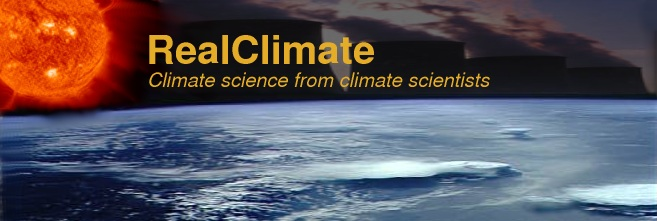#### Unforced variations

Filed under: — group @ 20 December 2009

Open thread for various climate science-related discussions. Suggestions for potential future posts are welcome.

(Continued here).

### 1,159 Responses to “Unforced variations”

1. 1151
wildlifer says:

Oh Boy!!!

Here’s one for you guys to take on, hot off of the presses:
No Rise of Atmospheric Carbon Dioxide Fraction in Past 160 Years, New Research Finds
http://www.sciencedaily.com/releases/2009/12/091230184221.htm

2. 1152
Brian Dodge says:

@ Ron Taylor — 31 December 2009 @ 10:37 AM
Don’t forget that the graphic you showed is for the anomaly; it’s warmer than normal, but still plenty cold, and ice is forming down the east coast of Greenland.
see http://igloo.atmos.uiuc.edu/cgi-bin/test/print.sh?fm=12&fd=29&fy=2009&sm=12&sd=29&sy=1994
Also, warmer water will evaporate more and become saltier and denser, tending to maintain the haline part of the thermohaline circulation. I predict this would also tend to drive heavier precipitation in Northern Europe, and will see if that’s true.

3. 1153
wildlifer says:

Gavin,
I did and the science-by-press-release leaves much to be desired.

They don’t fully explain what the science is talking about (a decrease/increase in absorption in the sinks not CO2, not increasing in the atmosphere), hence it’s gaining traction in the denialsphere.

4. 1154
Joseph says:

Earth is not a black body and even if it was 2 W/m2 in forcing is equivalent to 11 W/m2 in solar irradiance.

Thanks; you’re right. That’s 2 divided by (1 – albedo) times 4. In that case, 2 W/m^2 radiative forcing is quite a lot – way more than the data (e.g. Lean 2000) indicates.

BTW, I didn’t say Earth is a black body; only that (with all else being equal) its temperature is approximately proportional to the solar irradiance to the 1/4th power. It’s like saying that (with all else being equal) the equilibrium temperature depends on the CO2 concentration logarithmically – probably a much less accurate approximation.

5. 1155
Tom Dayton says:

wildlifer, there is a SkepticalScience post on that story: Is the airborne fraction of anthropogenic CO2 emissions increasing?

6. 1156
Patrick 027 says:

This could be useful to come back to later:

Climate sensitivity, response time, heat capacity (PS sorry for not using standard notation)

Reality is more complex then this, but considering:

a single heat capacity per unit area for the climate system,
C
(noting that the effective heat capacity tends to be larger for longer-period variations as the upper mixed ocean exchanges water with the deep ocean, the temperature changes penetrate into the land surface, etc.)

a constant blackbody radiation response, B, as a change in net upward LW flux per unit change in temperature T (this is a negative feedback, and including it, any stable climate equilibrium must have net negative feedback, but climate scientists usually take B as a given and speak of net feedbacks as being the rest of the feedbacks).

a constant non-B feedback F, which is a change in net upward LW radiation minus net downward SW radiation per unit change in T (note that positive F implies negative feedback). This feedback acts rapidly in response to changes in T relative to the T response to changes in forcing R. (This excludes some types of feedbacks.)

Then

where R’ and T’ are radiative forcing and temperature changes relative to an equilibrium baseline pair (R,T):

T’eq is the equilibrium T’

Let T’ – T’eq = T#

So dT’/dt = dT#/dt

dT’/dt = [R’-(B*T’)-(F*T’)]/C

[R’-(B*T’eq)-(F*T’eq)]/C = 0

R’ = (B+F)*T’eq

T’eq = R’/(B+F)

climate sensitivity = sens = T’eq/R’ = 1/(B+F)

“climate insensitivity” (can I introduce a term?) = Insens = R’/T’eq = B+F

dT’/dt
= [R’-(B+F)*T’]/C
= [R’-(B+F)*(T’eq + T#)]/C
= -(B+F)*T#/C

——-
For constant R’,

d(T’eq)/dt = 0 for constant R’, so

dT#/dt = [R’-(B*T’)-(F*T’)]/C
= [R’-(B+F)*(T’eq + T#)]/C
= -(B+F)*T#/C

Let
T’ = T’eq – A*exp(-t/r)
so
A*exp(-t/r) = T’eq – T’ = -T#

dT’/dt = dT#/dt = A/r * exp(-t/r)

A/r * exp(-t/r) = -(B+F)*T#/C = (B+F)/C * A*exp(-t/r)

1/r = (B+F)/C

r = C/(B+F) = C*sens = heat capacity * climate sensitivity

The time scale of climate response is proportional to climate sensitivity.

Notice also:

dT’/dt = R’/C; Starting at equilibrium, the initial rate of climate change is not sensitivity to climate sensitivity but is proportional to sudden forcing changes.

—–

Sinusoidal problem

Let R’ = A*cos(w*t)

T’eq = R’/(B+F) = A/(B+F) * cos(w*t)
T’ – T’eq = T#

Let T’ = G*cos(w*t) + H*sin(w*t)

dT’/dt = w*G*sin(w*t) – w*H*cos(w*t)

T# = [G – A/(B+F)]*cos(w*t) + H*sin(w*t)

dT’/dt = -(B+F)*T#/C

w*G*sin(w*t) = -(B+F)/C * H*sin(w*t)

and

w*H*cos(w*t) = (B+F)/C * [G – A/(B+F)]*cos(w*t)

w*G = -(B+F)/C * H

and

w*H = (B+F)/C * [G – A/(B+F)]

– w*G *C/(B+F) = H

and

H = (B+F)/(C*w) * [G – A/(B+F)]

– w*G *C/(B+F) = (B+F)/(C*w) * [G – A/(B+F)]

– [(C*w)/(B+F)]^2 = 1 – (A/G)*(B+F)

(A/G) = 1/(B+F) + (C*w)^2/(B+F)^3

G = A / [ 1/(B+F) + (C*w)^2/(B+F)^3 ]

H
= – G *(C*w)/(B+F)
= – A / [ 1/(C*w) + (C*w)/(B+F)^2 ]

(PS I hope this stays open for comments for a few more days)

7. 1157
Ian Love says:

re the reassessment of the HadCRUT records from ECMWF (comments #44, 163, 181) the paper by Simmonds et al. is now online for members

8. 1158
Alexandre says:

Another suggetion for future posts: positive feedbacks and runaway warming. To what extent do we know this? To what extent is it quantified? What could happen? What are the chances?

9. 1159
Jim Bouldin says:

Letter sent yesterday by a varied group of 40 scientists, to the American Farm Bureau Federation, questioning their stated position regarding the weight of evidence for human-induced climate change, and requesting a meeting with the AFBF director. The AFBF position letter states: “There is no generally agreed upon scientific assessment on the exact impact or extent of carbon emissions from human activities, their impact on past decades of warming, or how they will affect future climate changes. The UCS and Grist have stories.